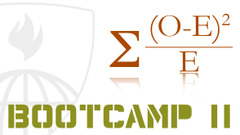## Mathematical Biostatistics Boot Camp 2

 所在平台: Coursera 课程类别: 统计和数据分析 授课老师： Brian CaffoExplore 1600+ online courses from top universities. Join Coursera today to learn data science, programming, business strategy, and more.#### 课程详情

This class presents fundamental concepts in data analysis and statistical inference, focusing on one and two independent samples. Students having taken this class should be able to summarize samples, perform relevant hypothesis tests and perform a collection of two sample comparisons. Classical non-parametric methods and discrete data analysis methods are discussed.  The class is taught at a master's of biostatistics introductory level and requires Mathematical Biostatistics Boot Camp 1 as a prerequisite.

#### 课程大纲

• Hypothesis Testing
• Power and sample size and two group tests
• Tests for binomial proportions
• Two sample binomial tests, delta method
• Fisher's exact tests, Chi-squared tests